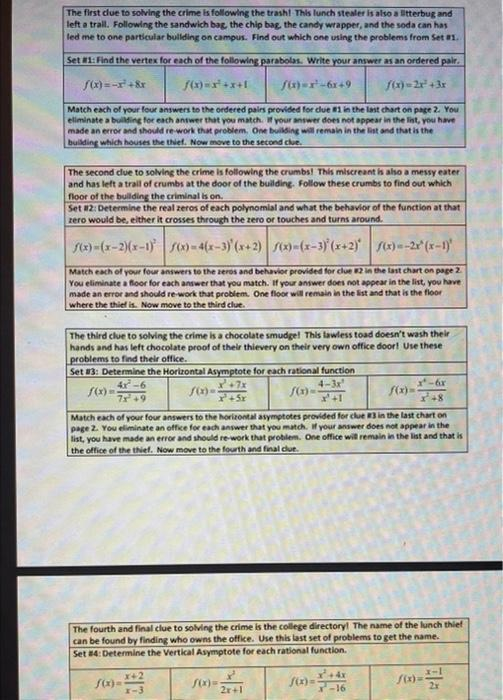Home / Expert Answers / Algebra / set-of-problems-nbsp-the-first-due-to-solving-the-crime-is-following-the-trashl-thls-lunch-stealer-pa506

# (Solved): set of problems  The first due to solving the crime is following the trashl-Thls lunch stealer ...

set of problemsThe first due to solving the crime is following the trashl-Thls lunch stealer is also a itterbug and left a trall. Following the sandwich boe, the chip bag, the candy wrapper, and the soda can has led me to one particular building on campus. Find out which one using the problems from Set a1. Match each of your four answers to the ordered pairs provided for clue in in the last chart on page 2 You elliminate a builfing for each antwer that you mateh. If Your answer does not oppear in the lint, you have made an error and thould re work that problem. One builsing will remain in the last and that is the bullaing which houses the thief. Now move to the second clue. The second due to solving the crime is following the crumbs! This miscreant in aho a messy eater and has left a trall of crumbs at the door of the bullding. Follow these crumbs to find out which floor of the bullding the criminal is on. Set il2: Determine the real zeror of each polynomial and what the behavior of the function at that zero would be, elther it crosses through the zero or touches and turns around. $$f(x)=(x-2)(x-1)^{2} \quad f(x)=4(x-3)^{4}(x+2) \quad f(x)=(x-3)^{2}(x+2)^{4} \quad f(x)=-2 x^{2}(x-1)^{4}$$ Match each of your four answers to the reros and behavior provided for clue 02 in the favt chart an page 2 . You eliminate a floor for each answer that you match. of your anfwer does not appear in the live, you have You eliminate a floor for each answer that you match. If your anwer does not appear in the lint, you have made an error and ahould re-work that problim. One floor will remain in the list and that is the floor where the thief is. Now move to the third elue. The fourth and final clue to solving the crime is the college directoryl The name of the lunch thief can be found by finding who owns the office. Use this last set of problems to get the name. Set #4: Determine the Vertical Asymptote for each rational function. $f(x)=\frac{x+2}{x-3} \quad f(x)=\frac{x^{3}}{2 x+1} \quad f(x)=\frac{x^{2}+4 x}{x^{2}-16} \quad f(x)=\frac{x-1}{2 x}$

We have an Answer from Expert